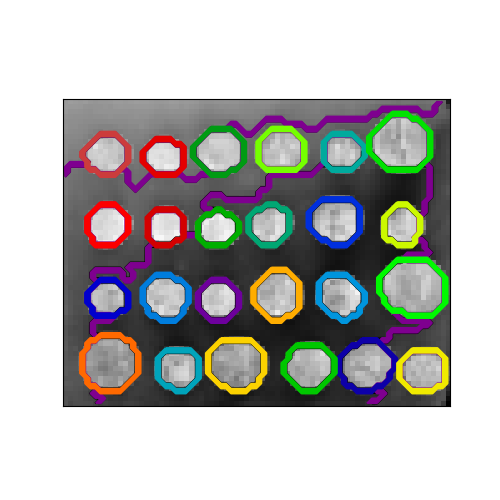# 5.2. 特征提取

## 5.2.1. 从字典类型加载特征

DictVectorizer 可用于将标准的Python字典（dict）对象列表的要素数组转换为 scikit-learn 估计器使用的 NumPy/SciPy 表示形式。

DictVectorizer 实现了 “one-of-K” 或 “one-hot” 编码，用于分类（也称为标称，离散）特征。分类功能是 “属性值” 对，其中该值被限制为不排序的可能性的离散列表（例如主题标识符，对象类型，标签，名称…）。

>>> measurements = [
...     {'city': 'Dubai', 'temperature': 33.},
...     {'city': 'London', 'temperature': 12.},
...     {'city': 'San Francisco', 'temperature': 18.},
... ]

>>> from sklearn.feature_extraction import DictVectorizer
>>> vec = DictVectorizer()

>>> vec.fit_transform(measurements).toarray()
array([[  1.,   0.,   0.,  33.],
[  0.,   1.,   0.,  12.],
[  0.,   0.,   1.,  18.]])

>>> vec.get_feature_names()
['city=Dubai', 'city=London', 'city=San Francisco', 'temperature']



DictVectorizer 也是对自然语言处理模型中训练序列分类器的有用的表示变换，通常通过提取围绕感兴趣的特定的词的特征窗口来工作。

>>> pos_window = [
...     {
...         'word-2': 'the',
...         'pos-2': 'DT',
...         'word-1': 'cat',
...         'pos-1': 'NN',
...         'word+1': 'on',
...         'pos+1': 'PP',
...     },
...     # in a real application one would extract many such dictionaries
... ]



>>> vec = DictVectorizer()
>>> pos_vectorized = vec.fit_transform(pos_window)
>>> pos_vectorized
<1x6 sparse matrix of type '<... 'numpy.float64'>'
with 6 stored elements in Compressed Sparse ... format>
>>> pos_vectorized.toarray()
array([[ 1.,  1.,  1.,  1.,  1.,  1.]])
>>> vec.get_feature_names()
['pos+1=PP', 'pos-1=NN', 'pos-2=DT', 'word+1=on', 'word-1=cat', 'word-2=the']



## 5.2.2. 特征哈希（相当于一种降维技巧）

FeatureHasher 是一种高速，低内存消耗的向量化方法，它使用了特征散列技术 ，或可称为 “散列法” （hashing trick）的技术。 代替在构建训练中遇到的特征的哈希表，如向量化所做的那样 FeatureHasher 将哈希函数应用于特征，以便直接在样本矩阵中确定它们的列索引。 结果是以牺牲可检测性为代价，提高速度和减少内存的使用; 哈希表不记得输入特性是什么样的，没有 inverse_transform 办法。

FeatureHasher 接受映射（如 Python 的 dict 及其在 collections 模块中的变体），使用键值对 (feature, value) 或字符串，具体取决于构造函数参数 input_type。 映射被视为 (feature, value) 对的列表，而单个字符串的隐含值为1，因此 ['feat1', 'feat2', 'feat3'] 被解释为 [('feat1', 1), ('feat2', 1), ('feat3', 1)]。 如果单个特征在样本中多次出现，相关值将被求和（所以 ('feat', 2)('feat', 3.5) 变为 ('feat', 5.5)）。 FeatureHasher 的输出始终是 CSR 格式的 scipy.sparse 矩阵。

def token_features(token, part_of_speech):
if token.isdigit():
yield "numeric"
else:
yield "token={}".format(token.lower())
yield "token,pos={},{}".format(token, part_of_speech)
if token.isupper():
yield "uppercase_initial"
if token.isupper():
yield "all_uppercase"
yield "pos={}".format(part_of_speech)



raw_X = (token_features(tok, pos_tagger(tok)) for tok in corpus)



hasher = FeatureHasher(input_type='string')
X = hasher.transform(raw_X)



### 5.2.2.1. 实现细节

FeatureHasher 使用签名的 32-bit 变体的 MurmurHash3。 因此导致（并且由于限制 scipy.sparse），当前支持的功能的最大数量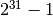.

## 5.2.3. 文本特征提取

### 5.2.3.1. 话语表示

• 令牌化（tokenizing） 对每个可能的词令牌分成字符串并赋予整数形的id，例如通过使用空格和标点符号作为令牌分隔符。
• 统计（counting） 每个词令牌在文档中的出现次数。
• 标准化（normalizing） 在大多数的文档 / 样本中，可以减少重要的次令牌的出现次数的权重。。

• 每个单独的令牌发生频率（标准化或不标准化）被视为一个特征
• 给定文档中所有的令牌频率向量被看做一个多元sample样本

### 5.2.3.3. 常见 Vectorizer 使用方法

CountVectorizer 在单个类中实现了 tokenization （词语切分）和 occurrence counting （出现频数统计）:

>>> from sklearn.feature_extraction.text import CountVectorizer



>>> vectorizer = CountVectorizer()
>>> vectorizer
CountVectorizer(analyzer=...'word', binary=False, decode_error=...'strict',
dtype=<... 'numpy.int64'>, encoding=...'utf-8', input=...'content',
lowercase=True, max_df=1.0, max_features=None, min_df=1,
ngram_range=(1, 1), preprocessor=None, stop_words=None,
strip_accents=None, token_pattern=...'(?u)\\b\\w\\w+\\b',
tokenizer=None, vocabulary=None)



>>> corpus = [
...     'This is the first document.',
...     'This is the second second document.',
...     'And the third one.',
...     'Is this the first document?',
... ]
>>> X = vectorizer.fit_transform(corpus)
>>> X
<4x9 sparse matrix of type '<... 'numpy.int64'>'
with 19 stored elements in Compressed Sparse ... format>



>>> analyze = vectorizer.build_analyzer()
>>> analyze("This is a text document to analyze.") == (
...     ['this', 'is', 'text', 'document', 'to', 'analyze'])
True



analyzer 在拟合过程中找到的每个 term（项）都会被分配一个唯一的整数索引，对应于 resulting matrix（结果矩阵）中的一列。此列的一些说明可以被检索如下:

>>> vectorizer.get_feature_names() == (
...     ['and', 'document', 'first', 'is', 'one',
...      'second', 'the', 'third', 'this'])
True

>>> X.toarray()
array([[0, 1, 1, 1, 0, 0, 1, 0, 1],
[0, 1, 0, 1, 0, 2, 1, 0, 1],
[1, 0, 0, 0, 1, 0, 1, 1, 0],
[0, 1, 1, 1, 0, 0, 1, 0, 1]]...)



>>> vectorizer.vocabulary_.get('document')
1



>>> vectorizer.transform(['Something completely new.']).toarray()
...
array([[0, 0, 0, 0, 0, 0, 0, 0, 0]]...)



>>> bigram_vectorizer = CountVectorizer(ngram_range=(1, 2),
...                                     token_pattern=r'\b\w+\b', min_df=1)
>>> analyze = bigram_vectorizer.build_analyzer()
>>> analyze('Bi-grams are cool!') == (
...     ['bi', 'grams', 'are', 'cool', 'bi grams', 'grams are', 'are cool'])
True



>>> X_2 = bigram_vectorizer.fit_transform(corpus).toarray()
>>> X_2
...
array([[0, 0, 1, 1, 1, 1, 1, 0, 0, 0, 0, 0, 1, 1, 0, 0, 0, 0, 1, 1, 0],
[0, 0, 1, 0, 0, 1, 1, 0, 0, 2, 1, 1, 1, 0, 1, 0, 0, 0, 1, 1, 0],
[1, 1, 0, 0, 0, 0, 0, 0, 1, 0, 0, 0, 1, 0, 0, 1, 1, 1, 0, 0, 0],
[0, 0, 1, 1, 1, 1, 0, 1, 0, 0, 0, 0, 1, 1, 0, 0, 0, 0, 1, 0, 1]]...)



>>> feature_index = bigram_vectorizer.vocabulary_.get('is this')
>>> X_2[:, feature_index]
array([0, 0, 0, 1]...)



### 5.2.3.4. Tf–idf 项加权

Tf表示术语频率，而 tf-idf 表示术语频率乘以转制文档频率: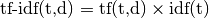.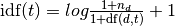,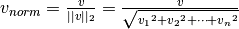.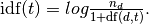TfidfTransformerTfidfVectorizersmooth_idf=False，将 “1” 计数添加到 idf 而不是 idf 的分母: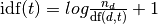>>> from sklearn.feature_extraction.text import TfidfTransformer
>>> transformer = TfidfTransformer(smooth_idf=False)
>>> transformer
TfidfTransformer(norm=...'l2', smooth_idf=False, sublinear_tf=False,
use_idf=True)



>>> counts = [[3, 0, 1],
...           [2, 0, 0],
...           [3, 0, 0],
...           [4, 0, 0],
...           [3, 2, 0],
...           [3, 0, 2]]
...
>>> tfidf = transformer.fit_transform(counts)
>>> tfidf
<6x3 sparse matrix of type '<... 'numpy.float64'>'
with 9 stored elements in Compressed Sparse ... format>

>>> tfidf.toarray()
array([[0.81940995, 0.        , 0.57320793],
[1.        , 0.        , 0.        ],
[1.        , 0.        , 0.        ],
[1.        , 0.        , 0.        ],
[0.47330339, 0.88089948, 0.        ],
[0.58149261, 0.        , 0.81355169]])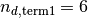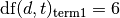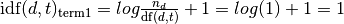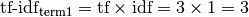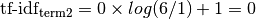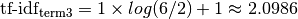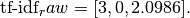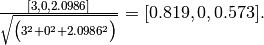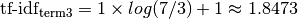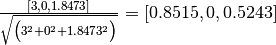:

>>> transformer = TfidfTransformer()
>>> transformer.fit_transform(counts).toarray()
array([[0.85151335, 0.        , 0.52433293],
[1.        , 0.        , 0.        ],
[1.        , 0.        , 0.        ],
[1.        , 0.        , 0.        ],
[0.55422893, 0.83236428, 0.        ],
[0.63035731, 0.        , 0.77630514]])



>>> transformer.idf_
array([1. ..., 2.25..., 1.84...])



>>> from sklearn.feature_extraction.text import TfidfVectorizer
>>> vectorizer = TfidfVectorizer()
>>> vectorizer.fit_transform(corpus)
...
<4x9 sparse matrix of type '<... 'numpy.float64'>'
with 19 stored elements in Compressed Sparse ... format>



### 5.2.3.5. 解码文本文件

scikit-learn 中的文本提取器知道如何解码文本文件， 但只有当您告诉他们文件的编码的情况下才行， CountVectorizer 才需要一个 encoding 参数。 对于现代文本文件，正确的编码可能是 UTF-8，因此它也是默认解码方式 (encoding="utf-8").

• 您可能可以使用 UNIX 命令 file 找出它一般使用什么样的编码。 Python chardet 模块附带一个名为 chardetect.py 的脚本，它会猜测具体的编码，尽管你不能依靠它的猜测是正确的。
• 你可以尝试 UTF-8 并忽略错误。您可以使用 bytes.decode(errors='replace') 对字节字符串进行解码，用无意义字符替换所有解码错误，或在向量化器中设置 decode_error='replace'. 这可能会损坏您的功能的有用性。
• 真实文本可能来自各种使用不同编码的来源，或者甚至以与编码的编码不同的编码进行粗略解码。这在从 Web 检索的文本中是常见的。Python 包 ftfy 可以自动排序一些解码错误类，所以您可以尝试将未知文本解码为 latin-1，然后使用 ftfy 修复错误。
• 如果文本的编码的混合，那么它很难整理分类（20个新闻组数据集的情况），您可以把它们回到简单的单字节编码，如 latin-1。某些文本可能显示不正确，但至少相同的字节序列将始终代表相同的功能。

>>> import chardet
>>> text1 = b"Sei mir gegr\xc3\xbc\xc3\x9ft mein Sauerkraut"
>>> text2 = b"holdselig sind deine Ger\xfcche"
>>> text3 = b"\xff\xfeA\x00u\x00f\x00 \x00F\x00l\x00\xfc\x00g\x00e\x00l\x00n\x00 \x00d\x00e\x00s\x00 \x00G\x00e\x00s\x00a\x00n\x00g\x00e\x00s\x00,\x00 \x00H\x00e\x00r\x00z\x00l\x00i\x00e\x00b\x00c\x00h\x00e\x00n\x00,\x00 \x00t\x00r\x00a\x00g\x00 \x00i\x00c\x00h\x00 \x00d\x00i\x00c\x00h\x00 \x00f\x00o\x00r\x00t\x00"
>>> decoded = [x.decode(chardet.detect(x)['encoding'])
...            for x in (text1, text2, text3)]
>>> v = CountVectorizer().fit(decoded).vocabulary_
>>> for term in v: print(v)



（根据 chardet 的版本，可能会返回第一个值错误的结果。） 有关 Unicode 和字符编码的一般介绍，请参阅Joel Spolsky的 绝对最低要求每个软件开发人员必须了解 Unicode.

### 5.2.3.7. 词语表示的限制

N-grams 可以帮助我们！而不是构建一个简单的unigrams集合 (n=1)，可能更喜欢一组二进制 (n=2)，其中计算连续字对。

>>> ngram_vectorizer = CountVectorizer(analyzer='char_wb', ngram_range=(2, 2))
>>> counts = ngram_vectorizer.fit_transform(['words', 'wprds'])
>>> ngram_vectorizer.get_feature_names() == (
...     [' w', 'ds', 'or', 'pr', 'rd', 's ', 'wo', 'wp'])
True
>>> counts.toarray().astype(int)
array([[1, 1, 1, 0, 1, 1, 1, 0],
[1, 1, 0, 1, 1, 1, 0, 1]])



>>> ngram_vectorizer = CountVectorizer(analyzer='char_wb', ngram_range=(5, 5))
>>> ngram_vectorizer.fit_transform(['jumpy fox'])
...
<1x4 sparse matrix of type '<... 'numpy.int64'>'
with 4 stored elements in Compressed Sparse ... format>
>>> ngram_vectorizer.get_feature_names() == (
...     [' fox ', ' jump', 'jumpy', 'umpy '])
True

>>> ngram_vectorizer = CountVectorizer(analyzer='char', ngram_range=(5, 5))
>>> ngram_vectorizer.fit_transform(['jumpy fox'])
...
<1x5 sparse matrix of type '<... 'numpy.int64'>'
with 5 stored elements in Compressed Sparse ... format>
>>> ngram_vectorizer.get_feature_names() == (
...     ['jumpy', 'mpy f', 'py fo', 'umpy ', 'y fox'])
True



### 5.2.3.8. 用哈希技巧矢量化大文本语料库

• 语料库越大，词汇量越大，使用的内存也越大.
• 拟合（fitting）需要根据原始数据集的大小等比例分配中间数据结构的大小.
• 构建词映射需要完整的传递数据集，因此不可能以严格在线的方式拟合文本分类器.
• pickling和un-pickling vocabulary 很大的向量器会非常慢（通常比pickling/un-pickling单纯数据的结构，比如同等大小的Numpy数组）.
• 将向量化任务分隔成并行的子任务很不容易实现，因为 vocabulary_ 属性要共享状态有一个细颗粒度的同步障碍：从标记字符串中映射特征索引与每个标记的首次出现顺序是独立的，因此应该被共享，在这点上并行worker的性能收到了损害，使他们比串行更慢。

>>> from sklearn.feature_extraction.text import HashingVectorizer
>>> hv = HashingVectorizer(n_features=10)
>>> hv.transform(corpus)
...
<4x10 sparse matrix of type '<... 'numpy.float64'>'
with 16 stored elements in Compressed Sparse ... format>



>>> hv = HashingVectorizer()
>>> hv.transform(corpus)
...
<4x1048576 sparse matrix of type '<... 'numpy.float64'>'
with 19 stored elements in Compressed Sparse ... format>



HashingVectorizer 还具有以下限制：

• 不能反转模型（没有inverse_transform方法），也无法访问原始的字符串表征，因为，进行mapping的哈希方法是单向本性。
• 没有提供了IDF权重，因为这需要在模型中引入状态。如果需要的话，可以在管道中添加 TfidfTransformer

### 5.2.3.10. 自定义矢量化器类

>>> def my_tokenizer(s):
...     return s.split()
...
>>> vectorizer = CountVectorizer(tokenizer=my_tokenizer)
>>> vectorizer.build_analyzer()(u"Some... punctuation!") == (
...     ['some...', 'punctuation!'])
True



• 预处理器: 可以将整个文档作为输入（作为单个字符串）的可调用，并返回文档的可能转换的版本，仍然是整个字符串。这可以用于删除HTML标签，小写整个文档等。
• tokenizer: 一个可从预处理器接收输出并将其分成标记的可调用函数，然后返回这些列表。
• 分析器: 一个可替代预处理程序和标记器的可调用程序。默认分析仪都会调用预处理器和刻录机，但是自定义分析仪将会跳过这个。 N-gram提取和停止字过滤在分析器级进行，因此定制分析器可能必须重现这些步骤。

（Lucene 用户可能会识别这些名称，但请注意，scikit-learn 概念可能无法一对一映射到 Lucene 概念上。）

• 如果文档由外部包进行预先标记，则将它们存储在文件（或字符串）中，令牌由空格分隔，并通过 analyzer=str.split
• Fancy 令牌级分析，如词干，词法，复合分割，基于词性的过滤等不包括在 scikit-learn 代码库中，但可以通过定制分词器或分析器来添加。这是一个 CountVectorizer, 使用 NLTK 的 tokenizer 和 lemmatizer: py

from nltk import word_tokenize
from nltk.stem import WordNetLemmatizer class LemmaTokenizer(object): ... def init(self): ... self.wnl = WordNetLemmatizer() ... def call(self, doc): ... return [self.wnl.lemmatize(t) for t in word_tokenize(doc)] ... vect = CountVectorizer(tokenizer=LemmaTokenizer())
（请注意，这不会过滤标点符号。） 例如，以下例子将英国的一些拼写变成美国拼写::py import re def to_british(tokens): ... for t in tokens: ... t = re.sub(r"(...)our$", r"\1or", t) ... t = re.sub(r"([bt])re$", r"\1er", t) ... t = re.sub(r"([iy])s(e$|ing|ation)", r"\1z\2", t) ... t = re.sub(r"ogue$", "og", t) ... yield t ... class CustomVectorizer(CountVectorizer): ... def build_tokenizer(self): ... tokenize = super().build_tokenizer() ... return lambda doc: list(to_british(tokenize(doc))) ... print(CustomVectorizer().build_analyzer()(u"color colour")) [...'color', ...'color']  用于其他样式的预处理; 例子包括 stemming, lemmatization, 或 normalizing numerical tokens, 后者说明如下:

## 5.2.4. 图像特征提取

### 5.2.4.1. 补丁提取

extract_patches_2d 函数从存储为二维数组的图像或沿着第三轴的颜色信息三维提取修补程序。 要从其所有补丁重建图像，请使用 reconstruct_from_patches_2d. 例如让我们使用3个彩色通道（例如 RGB 格式）生成一个 4x4 像素的图像:

>>> import numpy as np
>>> from sklearn.feature_extraction import image

>>> one_image = np.arange(4 * 4 * 3).reshape((4, 4, 3))
>>> one_image[:, :, 0]  # R channel of a fake RGB picture
array([[ 0,  3,  6,  9],
[12, 15, 18, 21],
[24, 27, 30, 33],
[36, 39, 42, 45]])

>>> patches = image.extract_patches_2d(one_image, (2, 2), max_patches=2,
...     random_state=0)
>>> patches.shape
(2, 2, 2, 3)
>>> patches[:, :, :, 0]
array([[[ 0,  3],
[12, 15]],

[[15, 18],
[27, 30]]])
>>> patches = image.extract_patches_2d(one_image, (2, 2))
>>> patches.shape
(9, 2, 2, 3)
>>> patches[4, :, :, 0]
array([[15, 18],
[27, 30]])



>>> reconstructed = image.reconstruct_from_patches_2d(patches, (4, 4, 3))
>>> np.testing.assert_array_equal(one_image, reconstructed)



PatchExtractor 以同样的方式类作品 extract_patches_2d, 只是它支持多种图像作为输入。它被实现为一个估计器，因此它可以在管道中使用。看到:

>>> five_images = np.arange(5 * 4 * 4 * 3).reshape(5, 4, 4, 3)
>>> patches = image.PatchExtractor((2, 2)).transform(five_images)
>>> patches.shape
(45, 2, 2, 3)



### 5.2.4.2. 图像的连接图

scikit-learn 中的几个估计可以使用特征或样本之间的连接信息。 例如，Ward聚类（层次聚类 层次聚类 ）可以聚集在一起，只有图像的相邻像素，从而形成连续的斑块: